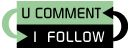## Tuesday, January 25, 2011

### Navigating (Searching) Collections

The Java collections framework includes the concept of NavigableSets / NavigableMaps. The principle behind these interfaces is that taking a SortedSet/SortedMap you can use a subset of it. Some examples:

Given the following set:

``````
@Before
public void setUp() {
set = new TreeSet();
set.addAll(Arrays.asList(1, 2, 3, 4, 6, 7, 8));
}
``````

The following is true:

``````
// Returns the least element in this set greater than or equal to the given element
assertEquals(Integer.valueOf(6), set.ceiling(5));
// Returns the greatest element in this set less than or equal to the given element
assertEquals(Integer.valueOf(4), set.floor(5));
// Returns the least element in this set strictly greater than the given element
assertEquals(Integer.valueOf(7), set.higher(6));
// Returns the greatest element in this set strictly less than the given element
assertEquals(Integer.valueOf(3), set.lower(4));

// Returns a view of the portion of this set whose elements are strictly less than toElement.
// Returns a view of the portion of this set whose elements are greater than or equal to fromElement.
assertTrue(set.tailSet(4).containsAll(Arrays.asList(4, 6, 7, 8)));
assertEquals(4, set.tailSet(4).size());
// Returns a view of the portion of this set whose elements range from fromElement, inclusive, to toElement, exclusive.
assertTrue(set.subSet(4, 8).containsAll(Arrays.asList(4, 6, 7)));
assertEquals(3, set.subSet(4, 8).size());
``````

Also, the subsets / submaps / "views" remain connected to the parent collection, so adding / removing to/from the parent collection updates them:

``````

// subsets remain connected
set.removeAll(Arrays.asList(1, 2));

// subsets remain connected
set.addAll(Arrays.asList(-1, 1, 2, 3, 4, 5));
``````

Finally, you manipulate the subsets and the result will be reflected in the original set (however if you try to add an out-of-range element, you will get an exception):

``````
assertTrue(set.containsAll(Arrays.asList(-1, 1, 2, 3, 4, 6, 7, 8)));
assertEquals(8, set.size());
``````

The implementation is very memory efficient, there is no copying of elements going on. One thing to consider is that by default these operations are not thread safe! Ie. if you generate two subsets of the same set and process them on two different threads, you must take care to properly synchronize the processing.

The complete source code can be found on Google Code under Public Domain or the BSD license.